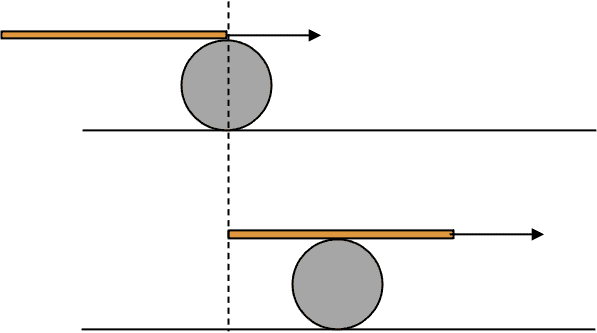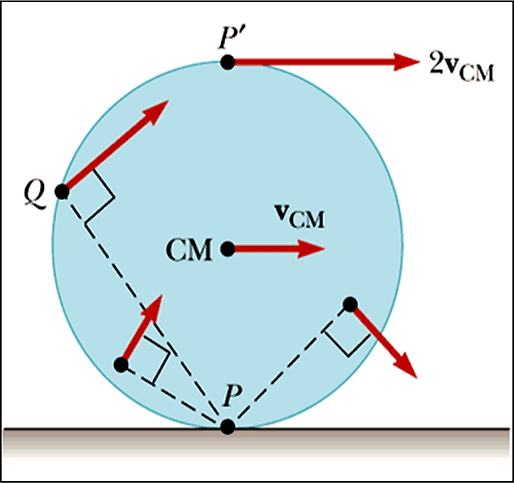# Rotational dynamics: Resolving forces

• wcjy
In summary, Frictional force between slope and cylinder produces no torque. Only torque comes from tension times diameter.

#### wcjy

Homework Statement
Consider a cylinder c of mass m = 10 kg and radius r 0.07 m rolls without slipping on slope H as shown below. The string is wrapped around cylinder c and does not stretch and does not slip on the cylinder. Assume the pulley to be massless.

(a) Explain very briefly why when the mass m = 2 kg moves by one meter, cylinder c moves vertically by 0.25m.

(b) Calculate the magnitude of a cm of cylinder c.
Relevant Equations
τ = I α
a = r α
Resolving the weight of the cylinder c, i get Mgcosθ (-y) and Mgsinθ (-x)

mgsinθ - Fs - T = ma ---(1) (where Fs is frictional force and T is tension)

τ = I α (where τ is torque and α is angular acceleration)
torque is produced by both tension and frictional force
(T-Fs) * r = 0.5 m r^2 α
a = r α
T- Fs = 0.5 m a
Fs = T - 0.5ma --- (2)

sub (2) into (1)
mgsinθ - T + 0.5ma -T = ma
mgsinθ -2T = 0.5ma
mgsingθ - 2(mg) = 0.5ma (where m in T is 2kg)
after putting in numbers,
a = 1.96m/s^2

ans: 0.426m/s^2

#### Attachments

•Screenshot 2020-10-02 211621.png
44 KB · Views: 159
Frictional force between slope and cylinder produces no torque.
Only torque comes from tension times diameter.

For an instantaneous snapshot of the configuration, which is what the problem asks for, it does not matter either you have a cylinder or a vertical bar with fulcrum at low end and tension acting on top end.

https://www.physicsforums.com/insights/explaining-rolling-motion/Last edited:
why though, frictional force doesn't act thru the cm thus it should by right cause torque. is my understanding flawed?

Yes.

soo am i right to use frictional force in my working?

Lnewqban said:
Frictional force between slope and cylinder produces no torque.
Well, that depends on the chosen axis.
If you take the cylinder's centre as axis then both string and friction produce torque, and to find the acceleration the value of I is ##\frac 12mr^2##.
But if you take the point of contact with the plane as axis then only the string exerts a torque and the MoI has to be adjusted according to the parallel axis theorem.

•wcjy
wait so now I am confused. am i right or am i wrong

•Delta2
wcjy said:
wait so now I am confused. am i right or am i wrong
When converting between rotational and linear motion and forces you must pay careful attention to signs.
Your linear acceleration equation for the cylinder takes a as positive down the slope. If you divide a by r then the rotational acceleration is therefore positive anticlockwise. But your torque equation takes clockwise as positive (T-Fs).

•wcjy
haruspex said:
When converting between rotational and linear motion and forces you must pay careful attention to signs.
Your linear acceleration equation for the cylinder takes a as positive down the slope. If you divide a by r then the rotational acceleration is therefore positive anticlockwise. But your torque equation takes clockwise as positive (T-Fs).
thanks for spotting out this mistake. However, after correcting, I still do not get the answer
i changed my equation to Fs - T= 0.5ma

Last edited:
wcjy said:
thanks for spotting out this mistake. However, after correcting, I still do not get the answer
Your next error was assuming the tension is just the weight of the hanging mass. If that were true, what would the acceleration of that mass be?

•wcjy
g?

wcjy said:
g?
What would the net force acting on the m=2kg mass be if the tension were mg?

0

why isn't the tension mg though? the weight of small mass is mg, therefore the tension in the string holding it should be mg due to N1L. since pulley is massless, the T should be = mg

wcjy said:
0
Right, so m would not accelerate.
What equation can you write for its acceleration? (Remember to use the result of the first part.)

sorry i lost you there.

wcjy said:
sorry i lost you there.
Write the vertical balance of forces, ΣF=ma, for mass m. How does its acceleration relate to the cylinder’s?

I'm not quite sure what you mean by vertical balance but I guess the small mass accelerates the same amount that the cylinder does.
i really don't understand why I am wrong, tension and friction acts in the (x) direction, and mgsin θ acts in the (-x) direction.

and the tension which is in the (x) direction is mg. the weight of small mass is mg, therefore the tension in the string holding it should be mg due to N1L. since pulley is massless, the T should be = mg

mgsin θ - T - fs = (m+M)a

wcjy said:
I'm not quite sure what you mean by vertical balance but I guess the small mass accelerates the same amount that the cylinder does.
i really don't understand why I am wrong, tension and friction acts in the (x) direction, and mgsin θ acts in the (-x) direction.

and the tension which is in the (x) direction is mg. the weight of small mass is mg, therefore the tension in the string holding it should be mg due to N1L. since pulley is massless, the T should be = mg

mgsin θ - T - fs = (m+M)a
As I explained, by vertical balance of forces I mean applying Newton’s second law, ΣF=ma, to the forces acting vertically on m and its resulting acceleration. You are assuming the tension is mg, but if you apply Newton's law you will find it is not.

Your assumption that the cylinder’s mass centre has the same acceleration as m is also incorrect. The first part of the question was designed to help you avoid that mistake. Did you do the first part?

•Lnewqban
ok sorry i had a big misconception. i finally understand what you are talking about the tension
T - mg = ma for small mass

Mgsin θ - Fs - mg = (M + m) a

Last edited:
wcjy said:
ok sorry i had a big misconception. i finally understand what you are talking about the tension
T - mg = ma for small mass

Mgsin θ - Fs - mg = (M + m) a
Yes, except that as I keep pointing out the two masses do not have the same linear acceleration. If mass m rises by 1m, how far does the cylinder roll down the slope?

can i just clarify for part a, the reason why its vert height drops by only 0.25m, is because the cylinder is wrapped around by the string, so when the small mass goes up by 1m, the distance cylinder travels diagonally downwards is 0.5m (since the string roll up on the cylinder). therefore vertically is 0.5*0.5 = 0.25m

wcjy said:
can i just clarify for part a, the reason why its vert height drops by only 0.25m, is because the cylinder is wrapped around by the string, so when the small mass goes up by 1m, the distance cylinder travels diagonally downwards is 0.5m (since the string roll up on the cylinder). therefore vertically is 0.5*0.5 = 0.25m
Yes. Now, we don't need to multiply by the second .5 because we are interested in the motion of the cylinder down the plane, not its vertical movement. But what does this tell you about the two accelerations?

the acceleration of the cylinder is half of small mass?

wcjy said:
the acceleration of the cylinder is half of small mass?
Yes.

so when i rewrite my equations,
T - mg = ma(small mass)
Mgsin θ - T - Fs = Ma(cylinder)
2a(cylinder) = a(small mass)

is this correct so far?

i got the answer. thanks for being patient and thanks for teaching me. <3

•Lnewqban
wcjy said:
Homework Statement:: Consider a cylinder c of mass m = 10 kg and radius r 0.07 m rolls without slipping on slope H as shown below. The string is wrapped around cylinder c and does not stretch and does not slip on the cylinder. Assume the pulley to be massless.

(a) Explain very briefly why when the mass m = 2 kg moves by one meter, cylinder c moves vertically by 0.25m.

(b) Calculate the magnitude of a cm of cylinder c.
Relevant Equations:: τ = I α
a = r α

---(1) (where Fs is frictional force and T
wcjy said:
can i just clarify for part a, the reason why its vert height drops by only 0.25m, is because the cylinder is wrapped around by the string, so when the small mass goes up by 1m, the distance cylinder travels diagonally downwards is 0.5m (since the string roll up on the cylinder). therefore vertically is 0.5*0.5 = 0.25m
I dun understand this why the cylinder will travel diagonally by 0.5

This thread is a bit more than 2 years old, but I will answer it because it is important for you to get this straight. Do a simple experiment as illustrated in the figure below.Place a can on a table and put a meter stick on the can with its tip above the center of the can. Place a small object on the table directly below the tip of the stick and the center of the table (where the dotted line is in the figure). Push the stick slowly so that it rolls without slipping on the can and the can rolls without slipping on the table. Stop when the 50 cm mark (half the length of the stick) is in contact with the can. Measure the length from the marker to the center of the can. It will be 50 cm. However, the tip of the rod has moved by 100 cm (the full length of the rod.)

Conclusion: When the stick advances by ##L##, the center of the can advances by ##L/2##. The same is true when, instead of a stick, you have a string unwrapping around the cylinder without slipping. That's because when the cylinder rolls without slipping on the table, the point in contact with the table is instantaneously at rest, the center of the circle is moving with speed ##v_c=\omega R## and the point at the top in contact with the rod is moving with speed ##v_t=\omega(2R)## which is twice as much as ##v_c##. Hence, the tip moves twice as fast as the center.

•erobz and Lnewqban